Three identical capacitors are given a charge Q each and they are then allowed to discharge through resistance R1, R2 and R3. Their charges, as a function of time shown in the graph below. The smallest of the three resistance is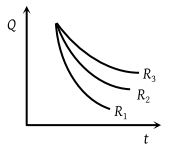(1) R3

(2) R2

(3) R1

(4) Cannot be predicted

Concept Questions :-

RC circuit
High Yielding Test Series + Question Bank - NEET 2020

Difficulty Level:

A condenser of 2μF capacitance is charged steadily from 0 to 5 Coulomb. Which of the following graphs correctly represents the variation of potential difference across its plates with respect to the charge on the condenser?

(1)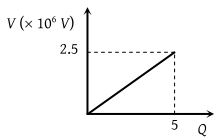(2)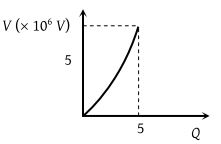(3)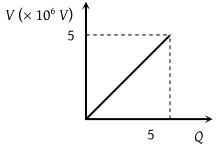(4)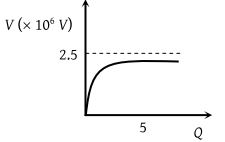Concept Questions :-

Capacitance
High Yielding Test Series + Question Bank - NEET 2020

Difficulty Level:

In an RC circuit while discharging, the graph of log i versus time is as shown by the dotted line in the diagram figure, where i is the current. When the value of the resistance is doubled, which of the solid curve best represents the variation of log i versus time ?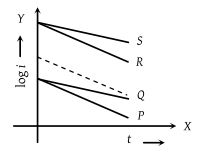(1) P

(2) Q

(3) R

(4) S

Concept Questions :-

RC circuit
High Yielding Test Series + Question Bank - NEET 2020

Difficulty Level:

Equipotential surfaces are shown in figure. Then the electric field strength will be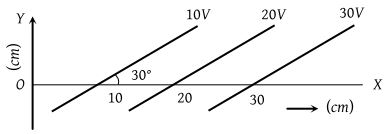(1) 100 Vm–1 along X-axis

(2) 100 Vm–1 along Y-axis

(3) 200 Vm–1 at an angle 120o with X-axis

(4) 50 Vm–1 at an angle 120o with X-axis

Concept Questions :-

Relation between field and potential
High Yielding Test Series + Question Bank - NEET 2020

Difficulty Level:

During charging a capacitor, the variation of potential V of the capacitor with time t is shown as :

1.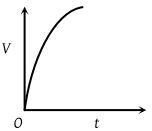2.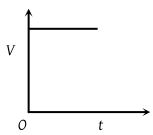3.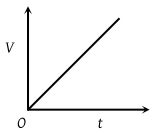4.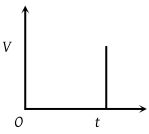Concept Questions :-

RC circuit
High Yielding Test Series + Question Bank - NEET 2020

Difficulty Level:

Figure (a) shows two capacitors connected in series and joined to a battery. The graph in figure (b) shows the variation in potential difference as one moves from left to right  on the branch containing the capacitors, if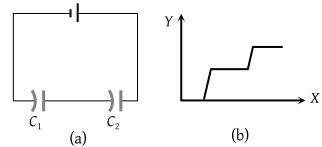(1) C1 > C2

(2) C1 = C2

(3) C1 < C2

(4) The information is not sufficient to decide the relation between C1 and ${C}_{2}$

Concept Questions :-

Combination of capactiors
High Yielding Test Series + Question Bank - NEET 2020

Difficulty Level:

Between the plates of a parallel plate capacitor a dielectric plate is introduced just to fill the space between the plates. The capacitor is charged and later disconnected from the battery. The dielectric plate is slowly drawn out of the capacitor parallel to the plates. The plot of the potential difference across the plates and the length of the dielectric plate drawn out is

(1)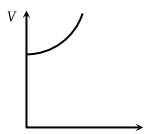(2)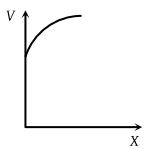(3)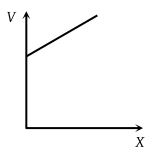(4)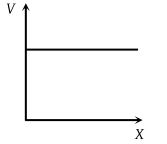Concept Questions :-

Combination of capactiors
High Yielding Test Series + Question Bank - NEET 2020

Difficulty Level:

What physical quantities may X and Y represent ? (Y represents the first mentioned quantity)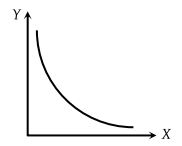(1) Pressure v/s temperature of a given gas (constant volume)

(2) Kinetic energy v/s velocity of a particle

(3) Capacitance v/s charge to give a constant potential

(4) Potential v/s capacitance to give a constant charge

Concept Questions :-

Capacitance
High Yielding Test Series + Question Bank - NEET 2020

Difficulty Level:

In a hollow spherical shell potential (V) changes with respect to distance (r) from centre

(1)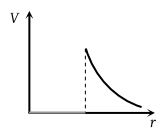(2)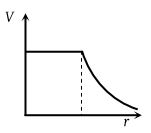(3)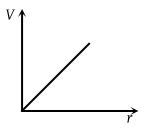(4)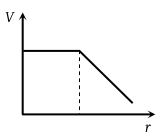Concept Questions :-

Electric potential
High Yielding Test Series + Question Bank - NEET 2020

Difficulty Level:

The figure gives the electric potential V as a function of distance through five regions on x-axis. Which of the following is true for the electric field E in these regions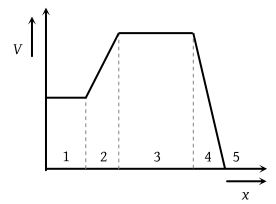(1) ${E}_{1}>{E}_{2}>{E}_{3}>{E}_{4}>{E}_{5}$

(2) ${E}_{1}={E}_{3}={E}_{5}$ and ${E}_{2}<{E}_{4}$

(3) ${E}_{2}={E}_{4}={E}_{5}$ and ${E}_{1}<{E}_{3}$

(4) ${E}_{1}<{E}_{2}<{E}_{3}<{E}_{4}<{E}_{5}$

Concept Questions :-

Relation between field and potential